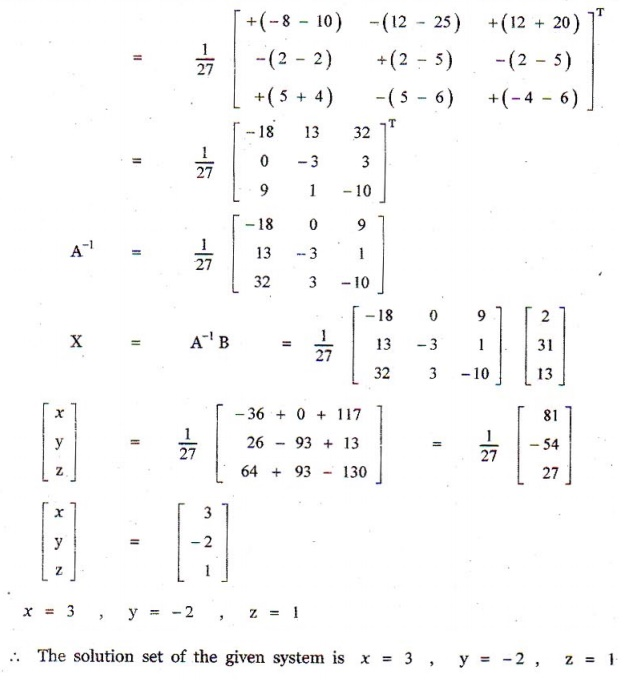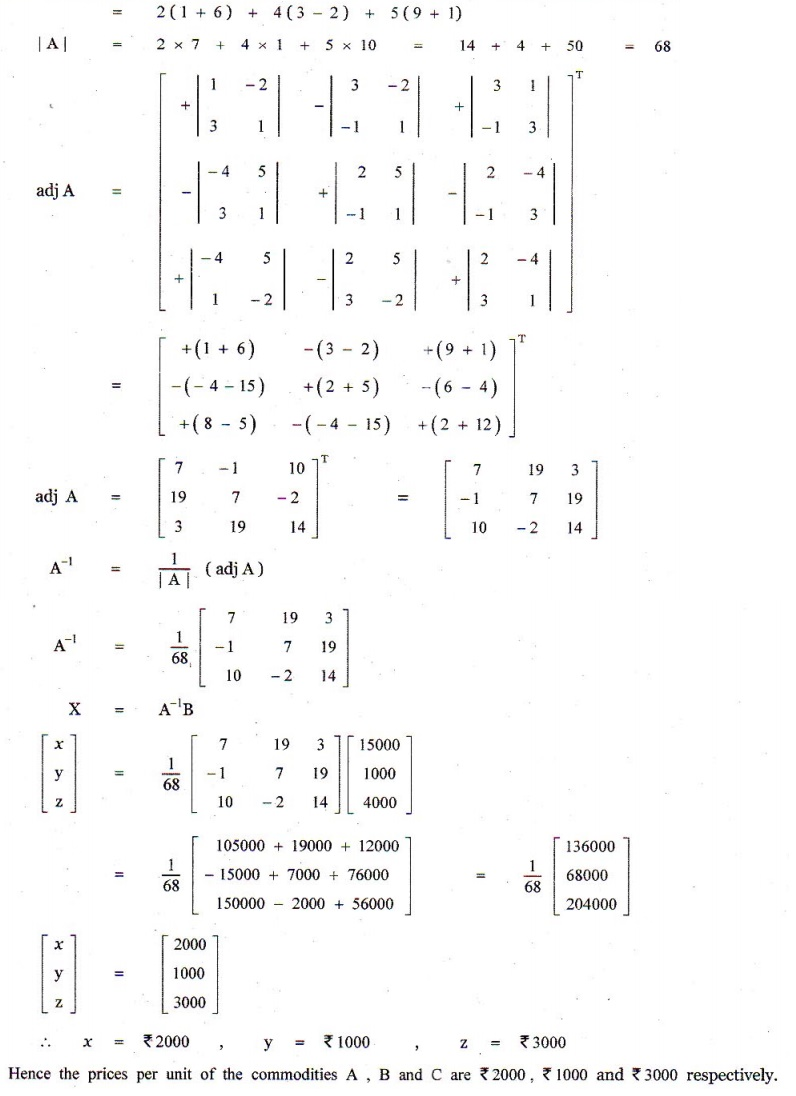Home | | Maths 12th Std | Exercise 1.3: Matrices linear equations by matrix inversion method

# Exercise 1.3: Matrices linear equations by matrix inversion method

Maths Book back answers and solution for Exercise questions - Solve the following system of linear equations by matrix inversion method:

EXERCISE 1.3

1. Solve the following system of linear equations by matrix inversion method:

(i) 2x + 5 y = -2, x + 2 y = -3

(ii) 2x - y = 8, 3x + 2 y = -2

(iii) 2x + 3y - z = 9, x + y + z = 9, 3x - y - z = -1

(iv) x + y + z - 2 = 0, 6x - 4 y + 5z - 31 = 0, 5x + 2 y + 2z = 132. Iffind the products AB and BA and hence solve the system of equations x + y + 2z = 1, 3x + 2 y + z = 7, 2x + y + 3z = 2.3. A man is appointed in a job with a monthly salary of certain amount and a fixed amount of annual increment. If his salary was â‚ą 19,800 per month at the end of the first month after 3 years of service and â‚ą 23,400 per month at the end of the first month after 9 years of service, find his starting salary and his annual increment. (Use matrix inversion method to solve the problem.)4. Four men and 4 women can finish a piece of work jointly in 3 days while 2 men and 5 women can finish the same work jointly in 4 days. Find the time taken by one man alone and that of one woman alone to finish the same work by using matrix inversion method.5. The prices of three commodities A, B and C are â‚ą x, y and z per units respectively. A person P purchases 4 units of B and sells two units of A and 5 units of C . Person Q purchases 2 units of C and sells 3 units of A and one unit of B . Person R purchases one unit of A and sells 3 unit of B and one unit of C . In the process, P,Q and R earn â‚ą 15,000, â‚ą 1,000 and â‚ą 4,000 respectively. Find the prices per unit of A, B and C . (Use matrix inversion method to solve the problem.)1. (i) x = -11, y = 4

(ii) x = 2, y = -4

(iii) x = 2, y = 3, z = 4

(iv) x = 3, y = -2, z = 1

2. x = 2, y = 1, z = -1

3. â‚ą 18000, â‚ą 600

4. 18 days, 36 days

5. â‚ą 2000, â‚ą 1000, â‚ą 3000

Tags : Problem Questions with Answer, Solution , 12th Mathematics : UNIT 1 : Applications of Matrices and Determinants
Study Material, Lecturing Notes, Assignment, Reference, Wiki description explanation, brief detail
12th Mathematics : UNIT 1 : Applications of Matrices and Determinants : Exercise 1.3: Matrices linear equations by matrix inversion method | Problem Questions with Answer, Solution